Skip to content
Related Articles
Octave – Basics of Plotting Data
• Last Updated : 01 Aug, 2020

Octave has some in-built functions for visualizing the data. Few simple plots can give us a better way to understand our data. whenever we perform a learning algorithm on an Octave environment, we can get a better sense of that algorithm and analyze it. Octave has lots of simple tools that we can use for a better understanding of our algorithm.
In this tutorial, we are going to learn how to plot data for better visualization and understanding it in the Octave environment.

Example 1 : Plotting a sine wave using the `plot()` and and `sin()` function:

 `% var_x for the y-axis``var_x = [0:0.01:1];`` ` `% var_y for the y-axis``var_y = sin(4 * pi * var);`` ` `% plotting the graph``plot(var_x, var_y);`

Output :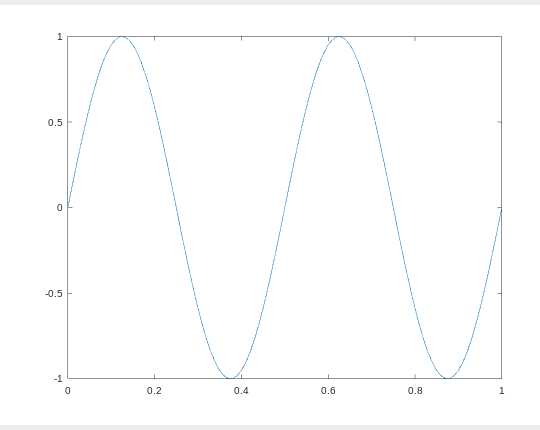Example 2 : Plotting a cosine wave using the `plot()` and and `cos()` function:

 `% var_x for the y-axis``var_x = [0:0.01:1];`` ` `% var_y for the y-axis``var_y = cos(3 * pi * var);`` ` `% plotting the graph``plot(var_x, var_y);`

Output :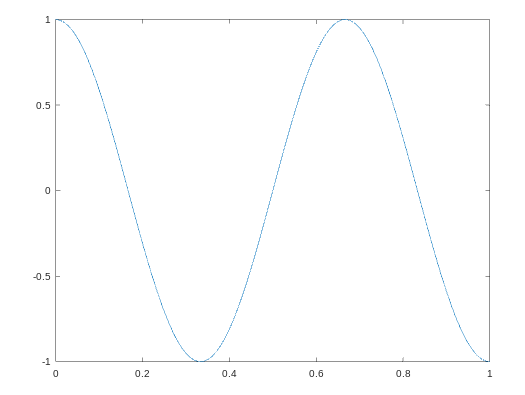Example 3 : We can plot, one plot over another plot by holding the previous plot with the `hold on` command.

 `% declaring variable var_x``var_x = [0:0.01:1];`` ` `% declaring variable var_y1``var_y1 = sin(4 * pi * var);`` ` `% declaring variable var_y2``var_y2 = cos(3 * pi * var);`` ` `% plot var_x with var_y1``plot(var_x, var_y1);`` ` `% hold the above plot or figure``hold on;`` ` `% plot var with var_y2 with red color``plot(var_x, var_y2, ``'r'``);`

Output :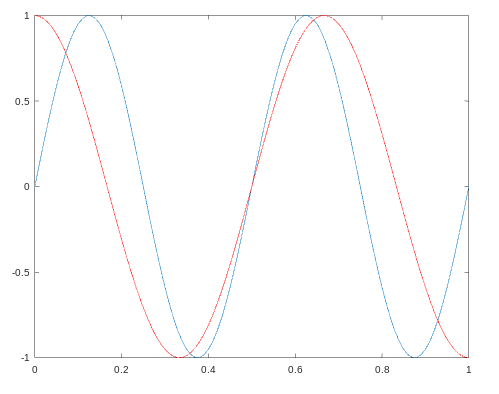Example 4 : We can add labels for the x-axis and the y-axis along with the `legends` and `title` with the below code.

 `% declaring variable var_x``var_x = [0:0.01:1];`` ` `% declaring variable var_y1``var_y1 = sin(4 * pi * var);`` ` ` ` `% declaring variable var_y2``var_y2 = cos(3 * pi * var);`` ` `% plot var_x with var_y1``plot(var_x, var_y1);`` ` `% hold the above plot or figure``hold on;`` ` `% plot var with var_y2 with red color``plot(var_x, var_y2, ``'r'``);`` ` `% adding label to the x-axis``xlabel(``'time'``);`` ` `% adding lable to the y-axis``ylabel(``'value'``);`` ` `% adding title for the plot``title(``'my first plot'``);`` ` `% add legends for these 2 curves``legend(``'sin'``, ``'cos'``);`

Output :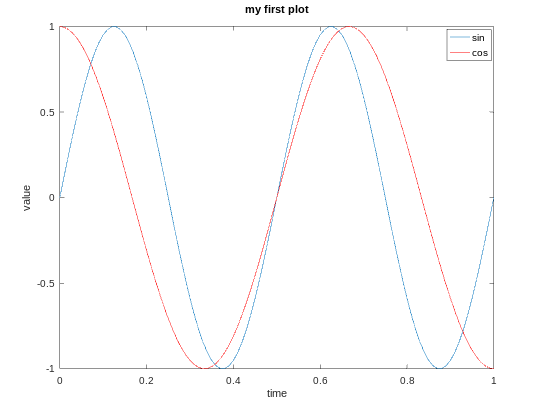Example 5 : We can also plot data on different figures.

 `% declaring variable var_x``var_x = [0:0.01:1];``  ` `% declaring variable var_y1``var_y1 = sin(4 * pi * var);``  ` `% declaring variable var_y2``var_y2 = cos(3 * pi * var);`` ` `% plot var_x and var_y1 on figure 1``figure(1); ``plot(var_x,var_y);`` ` `% plot var_x and var_y2 on figure 2``figure(2); ``plot(var_x,var_y2);`

Output :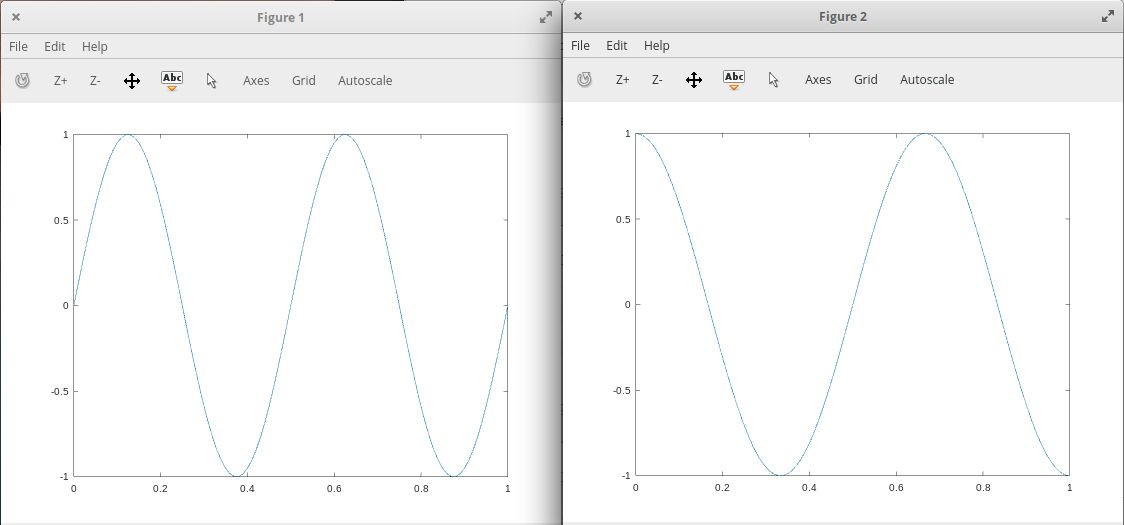Example 6 : We can divide a figure into a m x n grid using the `subplot()` function. In the below code the first 2 parameter shows m and n and 3rd parameter is the grid count from top to left.

 `% var_x for the y-axis``var_x = [0:0.01:1];``  ` `% var_y for the y-axis``var_y = sin(4 * pi * var);`` ` `% plot the var_x and var_y on a 3x3 grid ``% at 4 position counting from top to left``subplot(3, 3, 4), plot(var_x, var_y);`

Output :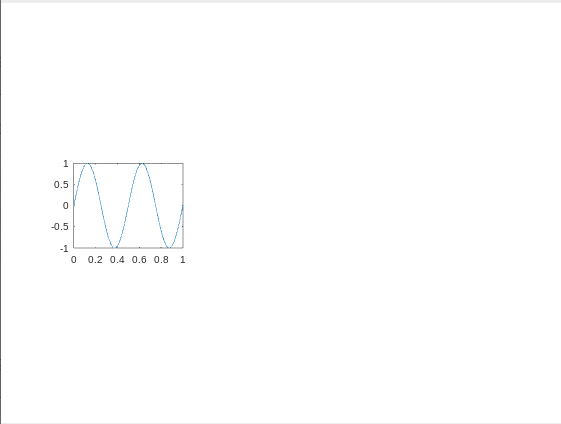Example 7 : We can change the `axis` values of any plot using the `axis()` function.

 `% declaring variable var_x``var_x = [0:0.01:1];``  ` `% declaring variable var_y1``var_y1 = sin(4 * pi * var);``  ` `% declaring variable var_y2``var_y2 = cos(3 * pi * var);``  ` `% plot var_x with var_y1``plot(var_x, var_y1);``  ` `% hold the above plot or figure``hold on;``  ` `% plot var with var_y2 with red color``plot(var_x, var_y2, ``'r'``);`` ` `% adding label to the x-axis``xlabel(``'time'``);`` ` `% adding lable to the y-axis``ylabel(``'value'``);``  ` `% adding title for the plot``title(``'my first plot'``);``  ` `% add legends for these 2 curves``legend(``'sin'``, ``'cos'``);`` ` `% first 2 parameter sets the x-axis ``% and next 2 will set the y-axis``axis([0.5 1 -1 1])`

Here the first 2 parameters shows the range of the x-axis and the next 2 parameters shows the range of the y-axis.
Output :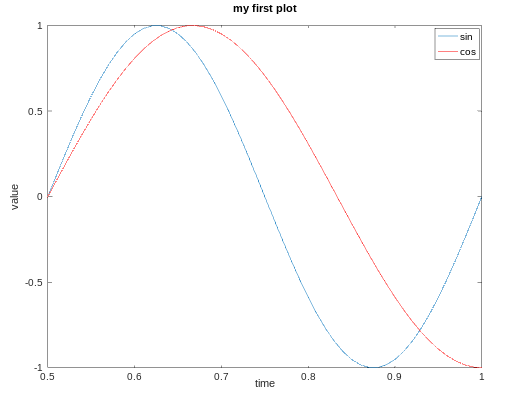Example 8 : We can save our plots in our present working directory :

 `print -dpng ``'plot.png'`

In order to print this plot at our desired location, we can use cd with it as shown below :

 `cd ``'/home/dikshant/Documents'``; print -dpng ``'plot.png'`

We can close a figure/plot using the `close `command.

Example 9 : We can visualize a matrix using the `imagesc(`) function.

 `% creating a 10x10 magic matrix``matrix = magic(10)`` ` `% plot the matrix``imagesc(matrix)`

Output :

```matrix =

92    99     1     8    15    67    74    51    58    40
98    80     7    14    16    73    55    57    64    41
4    81    88    20    22    54    56    63    70    47
85    87    19    21     3    60    62    69    71    28
86    93    25     2     9    61    68    75    52    34
17    24    76    83    90    42    49    26    33    65
23     5    82    89    91    48    30    32    39    66
79     6    13    95    97    29    31    38    45    72
10    12    94    96    78    35    37    44    46    53
11    18   100    77    84    36    43    50    27    59
```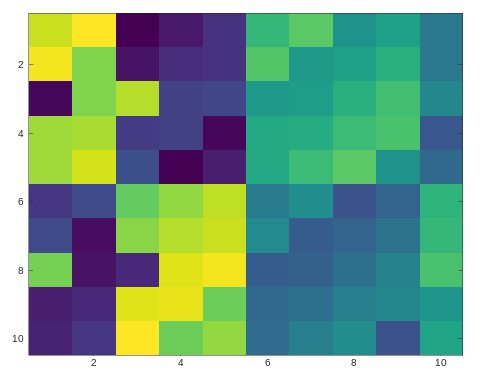The above plot is of 10×10 grid, each grid represents a value with a color. The same color value results in the same color.

We can also make a color bar with this plot to see which value corresponds to which color using the `colorbar` command. We can use multiple commands at a time by separating them with a comma(,) in Octave environment.

 `% creating a 10x10 magic matrix``matrix = magic(10)`` ` `% plot this matrix with showing colorbar on the right of it``imagesc(matrix), colorbar;`

Output :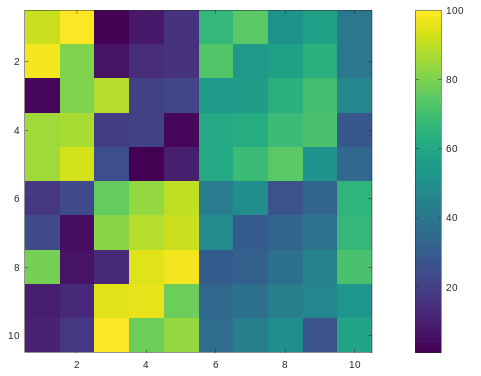Drawing the magic square with a gray-scale colormap :

 `% creating a 10x10 magic matrix``matrix = magic(10)`` ` `% plot this matrix with colorbar and gray colormap``imagesc(matrix), colorbar, colormap gray;`

Output :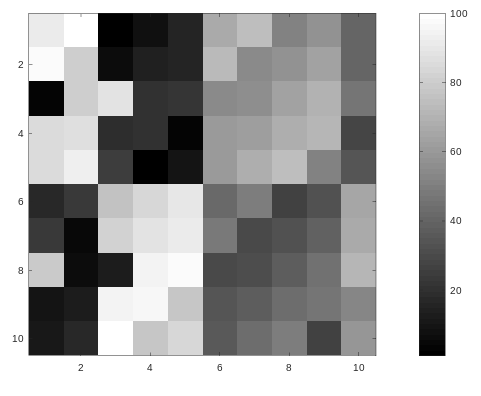My Personal Notes arrow_drop_up